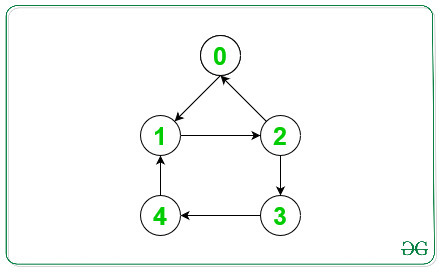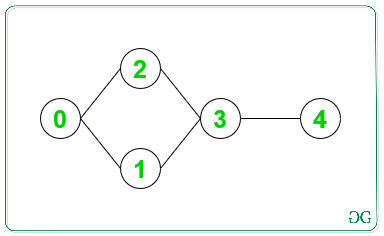# Convert undirected connected graph to strongly connected directed graph

Given an undirected graph of N vertices and M edges, the task is to assign directions to the given M Edges such that the graph becomes Strongly Connected Components. If a graph cannot be converted into Strongly Connected Components then print “-1”.
Examples:

Input: N = 5, Edges[][] = { { 0, 1 }, { 0, 2 }, { 1, 2 }, { 1, 4 }, { 2, 3 }, { 3, 4 } }
Output:
0->1
2->0
4->1
3->4
2->3
1->2
Explanation:
Below is the assigned edges to the above undirected graph:Input: N = 5, Edges[][] = { { 0, 1 }, { 0, 2 }, { 1, 3 }, { 2, 3 }, { 3, 4 } }
Output: -1
Explanation:
Below is the graph for the above information:Since there is a bridge present in the above-undirected graph. Therefore, this graph can’t be converted into SCCs.

Approach: We know that in any directed graph is said to be in Strongly Connected Components(SCCs) iff all the vertices of the graph are a part of some cycle. The given undirected graph doesn’t form SCCs if and only if the graph contains any bridges in it. Below are the steps:

• We will use an array mark[] to store the visited node during DFS Traversal, order[] to store the index number of the visited node, and bridge_detect[] to store any bridge present in the given graph.
• Start the DFS Traversal from vertex 1.
• Traverse the Adjacency list of current Node and do the following:
• If any edges are traverse again while any DFS call then ignore that edges.
• If the order of child Node(Node u) is greater than the order of parent node(node v), then ignore this current edges as as Edges(v, u) is already processed.
• If any Back Edge is found then update the Bridge Edges of the current parent node(node v) as:
``` bridge_detect[v] = min(order[u], bridge_detect[v]);

```
• Else do the DFS Traversal for the current child node and repeat step 3 for the current node.
• Update the bridges detect after DFS call for the current node as:
```bridge_detect[v] = min(bridge_detect[u], bridge_detect[v])

```
• Store the current pair of Edges(v, u) as directed Edges from Node v to Node u in an array of pairs(say arr[][]).
• If there is any bridge present in the given graph then print “-1”.
• Else print the directed Edges stored in arr[][].

Below is the implementation of the above approach:

## C++

 `// C++ program for the above approach`   `#include ` `using` `namespace` `std;`   `// To store the assigned Edges` `vector > ans;`   `// Flag variable to check Bridges` `int` `flag = 1;`   `// Function to implement DFS Traversal` `int` `dfs(vector<``int``> adj[],` `        ``int``* order, ``int``* bridge_detect,` `        ``bool``* mark, ``int` `v, ``int` `l)` `{`   `    ``// Mark the current node as visited` `    ``mark[v] = 1;`   `    ``// Update the order of node v` `    ``order[v] = order[l] + 1;`   `    ``// Update the bridge_detect for node v` `    ``bridge_detect[v] = order[v];`   `    ``// Traverse the adjacency list of` `    ``// Node v` `    ``for` `(``int` `i = 0; i < adj[v].size(); i++) {` `        ``int` `u = adj[v][i];`   `        ``// Ignores if same edge is traversed` `        ``if` `(u == l) {` `            ``continue``;` `        ``}`   `        ``// Ignores the edge u --> v as` `        ``// v --> u is already processed` `        ``if` `(order[v] < order[u]) {` `            ``continue``;` `        ``}`   `        ``// Finds a back Edges, cycle present` `        ``if` `(mark[u]) {`   `            ``// Update the bridge_detect[v]` `            ``bridge_detect[v]` `                ``= min(order[u],` `                      ``bridge_detect[v]);` `        ``}`   `        ``// Else DFS traversal for current` `        ``// node in the adjacency list` `        ``else` `{`   `            ``dfs(adj, order, bridge_detect,` `                ``mark, u, v);` `        ``}`   `        ``// Update the bridge_detect[v]` `        ``bridge_detect[v]` `            ``= min(bridge_detect[u],` `                  ``bridge_detect[v]);`   `        ``// Store the current directed Edge` `        ``ans.push_back(make_pair(v, u));` `    ``}`   `    ``// Condition for Bridges` `    ``if` `(bridge_detect[v] == order[v]` `        ``&& l != 0) {` `        ``flag = 0;` `    ``}`   `    ``// Return flag` `    ``return` `flag;` `}`   `// Function to print the direction` `// of edges to make graph SCCs` `void` `convert(vector<``int``> adj[], ``int` `n)` `{`   `    ``// Arrays to store the visited,` `    ``// bridge_detect and order of` `    ``// Nodes` `    ``int` `order[n] = { 0 };` `    ``int` `bridge_detect[n] = { 0 };` `    ``bool` `mark[n];`   `    ``// Intialise marks[] as false` `    ``memset``(mark, ``false``, ``sizeof``(mark));`   `    ``// DFS Traversal from vertex 1` `    ``int` `flag = dfs(adj, order,` `                   ``bridge_detect,` `                   ``mark, 1, 0);`   `    ``// If flag is zero, then Bridge` `    ``// is present in the graph` `    ``if` `(flag == 0) {` `        ``cout << ``"-1"``;` `    ``}`   `    ``// Else print the direction of` `    ``// Edges assigned` `    ``else` `{` `        ``for` `(``auto``& it : ans) {` `            ``cout << it.first << ``"->"` `                 ``<< it.second << ``'\n'``;` `        ``}` `    ``}` `}`   `// Function to create graph` `void` `createGraph(``int` `Edges[],` `                 ``vector<``int``> adj[],` `                 ``int` `M)` `{`   `    ``// Traverse the Edges` `    ``for` `(``int` `i = 0; i < M; i++) {`   `        ``int` `u = Edges[i];` `        ``int` `v = Edges[i];`   `        ``// Push the edges in an` `        ``// adjacency list` `        ``adj[u].push_back(v);` `        ``adj[v].push_back(u);` `    ``}` `}`   `// Driver Code` `int` `main()` `{` `    ``// N vertices and M Edges` `    ``int` `N = 5, M = 6;` `    ``int` `Edges[M]` `        ``= { { 0, 1 }, { 0, 2 },` `            ``{ 1, 2 }, { 1, 4 },` `            ``{ 2, 3 }, { 3, 4 } };`   `    ``// To create Adjacency List` `    ``vector<``int``> adj[N];`   `    ``// Create an undirected graph` `    ``createGraph(Edges, adj, M);`   `    ``// Function Call` `    ``convert(adj, N);` `    ``return` `0;` `}`

## Java

 `// Java program for the above approach` `import` `java.util.*;` `import` `java.lang.*;`   `class` `GFG{` `    `  `// To store the assigned Edges` `static` `ArrayList<``int``[]> ans;` ` `  `// Flag variable to check Bridges` `static` `int` `flag = ``1``;` ` `  `// Function to implement DFS Traversal` `static` `int` `dfs(ArrayList> adj,` `               ``int``[] order, ``int``[] bridge_detect,` `               ``boolean``[] mark, ``int` `v, ``int` `l)` `{` `    `  `    ``// Mark the current node as visited` `    ``mark[v] = ``true``;` `    `  `    ``// Update the order of node v` `    ``order[v] = order[l] + ``1``;` ` `  `    ``// Update the bridge_detect for node v` `    ``bridge_detect[v] = order[v];` ` `  `    ``// Traverse the adjacency list of` `    ``// Node v` `    ``for``(``int` `i = ``0``; i < adj.get(v).size(); i++)` `    ``{` `        ``int` `u = adj.get(v).get(i);` ` `  `        ``// Ignores if same edge is traversed` `        ``if` `(u == l)` `        ``{` `            ``continue``;` `        ``}` ` `  `        ``// Ignores the edge u --> v as` `        ``// v --> u is already processed` `        ``if` `(order[v] < order[u])` `        ``{` `            ``continue``;` `        ``}` ` `  `        ``// Finds a back Edges, cycle present` `        ``if` `(mark[u]) ` `        ``{` `            `  `            ``// Update the bridge_detect[v]` `            ``bridge_detect[v] = Math.min(order[u],` `                                ``bridge_detect[v]);` `        ``}` ` `  `        ``// Else DFS traversal for current` `        ``// node in the adjacency list` `        ``else` `        ``{` `            ``dfs(adj, order, bridge_detect,` `                ``mark, u, v);` `        ``}` ` `  `        ``// Update the bridge_detect[v]` `        ``bridge_detect[v] = Math.min(bridge_detect[u],` `                                    ``bridge_detect[v]);` ` `  `        ``// Store the current directed Edge` `        ``ans.add(``new` `int``[]{v, u});` `    ``}` ` `  `    ``// Condition for Bridges` `    ``if` `(bridge_detect[v] == order[v] && l != ``0``)` `    ``{` `        ``flag = ``0``;` `    ``}` `    `  `    ``// Return flag` `    ``return` `flag;` `}` ` `  `// Function to print the direction` `// of edges to make graph SCCs` `static` `void` `convert(ArrayList> adj, ` `                    ``int` `n)` `{` `    `  `    ``// Arrays to store the visited,` `    ``// bridge_detect and order of` `    ``// Nodes` `    ``int``[] order = ``new` `int``[n];` `    ``int``[] bridge_detect = ``new` `int``[n];` `    ``boolean` `mark[] = ``new` `boolean``[n];` `    `  `    ``// DFS Traversal from vertex 1` `    ``int` `flag = dfs(adj, order,` `                   ``bridge_detect,` `                   ``mark, ``1``, ``0``);` ` `  `    ``// If flag is zero, then Bridge` `    ``// is present in the graph` `    ``if` `(flag == ``0``) ` `    ``{` `        ``System.out.print(``"-1"``);` `    ``}` ` `  `    ``// Else print the direction of` `    ``// Edges assigned` `    ``else` `    ``{` `        ``for``(``int``[] it : ans)` `        ``{` `            ``System.out.println(it[``0``] + ``"->"` `+ ` `                               ``it[``1``]);` `        ``}` `    ``}` `}` ` `  `// Function to create graph` `static` `void` `createGraph(``int` `Edges[][],` `                        ``ArrayList> adj,` `                        ``int` `M)` `{` `    `  `    ``// Traverse the Edges` `    ``for``(``int` `i = ``0``; i < M; i++) ` `    ``{` `        ``int` `u = Edges[i][``0``];` `        ``int` `v = Edges[i][``1``];` `        `  `        ``// Push the edges in an` `        ``// adjacency list` `        ``adj.get(u).add(v);` `        ``adj.get(v).add(u);` `    ``}` `}`   `// Driver code` `public` `static` `void` `main(String[] args)` `{` `    `  `    ``// N vertices and M Edges` `    ``int` `N = ``5``, M = ``6``;` `    `  `    ``int` `Edges[][] = { { ``0``, ``1` `}, { ``0``, ``2` `},` `                      ``{ ``1``, ``2` `}, { ``1``, ``4` `},` `                      ``{ ``2``, ``3` `}, { ``3``, ``4` `} };` `    `  `    ``// To create Adjacency List` `    ``ArrayList> adj = ``new` `ArrayList<>();` `    ``ans = ``new` `ArrayList<>();` `    `  `    ``for``(``int` `i = ``0``; i < N; i++)` `        ``adj.add(``new` `ArrayList<>());` `    `  `    ``// Create an undirected graph` `    ``createGraph(Edges, adj, M);` `    `  `    ``// Function Call` `    ``convert(adj, N);` `}` `}`   `// This code is contributed by offbeat`

## Python3

 `# Python3 program for ` `# the above approach`   `# To store the assigned ` `# Edges` `ans ``=` `[]` ` `  `# Flag variable to ` `# check Bridges` `flag ``=` `1``;` ` `  `# Function to implement ` `# DFS Traversal` `def` `dfs(adj, order, ` `        ``bridge_detect, ` `        ``mark, v, l):` `    `  `    ``global` `flag` `    `  `    ``# Mark the current ` `    ``# node as visited` `    ``mark[v] ``=` `1``;` ` `  `    ``# Update the order of ` `    ``# node v` `    ``order[v] ``=` `order[l] ``+` `1``;` ` `  `    ``# Update the bridge_detect ` `    ``# for node v` `    ``bridge_detect[v] ``=` `order[v];` ` `  `    ``# Traverse the adjacency list of` `    ``# Node v` `    ``for` `i ``in` `range``(``len``(adj[v])):        ` `        ``u ``=` `adj[v][i];` ` `  `        ``# Ignores if same edge ` `        ``# is traversed` `        ``if` `(u ``=``=` `l):` `            ``continue``;       ` ` `  `        ``# Ignores the edge u --> v as` `        ``# v --> u is already processed` `        ``if` `(order[v] < order[u]):` `            ``continue``;        ` ` `  `        ``# Finds a back Edges, ` `        ``# cycle present` `        ``if` `(mark[u]):` ` `  `            ``# Update the bridge_detect[v]` `            ``bridge_detect[v] ``=` `min``(order[u],` `                                  ``bridge_detect[v]);` `        `  `        ``# Else DFS traversal for current` `        ``# node in the adjacency list` `        ``else``:` ` `  `            ``dfs(adj, order, ` `                ``bridge_detect,` `                ``mark, u, v);        ` ` `  `        ``# Update the bridge_detect[v]` `        ``bridge_detect[v] ``=` `min``(bridge_detect[u],` `                              ``bridge_detect[v]);` ` `  `        ``# Store the current ` `        ``# directed Edge` `        ``ans.append([v, u]);` ` `  `    ``# Condition for Bridges` `    ``if` `(bridge_detect[v] ``=``=` `        ``order[v] ``and` `l !``=` `0``):` `        ``flag ``=` `0``;` `    `  `    ``# Return flag` `    ``return` `flag;` ` `  `# Function to print the ` `# direction of edges to ` `# make graph SCCs` `def` `convert(adj, n):` ` `  `    ``# Arrays to store the visited,` `    ``# bridge_detect and order of` `    ``# Nodes` `    ``order ``=` `[``0` `for` `i ``in` `range``(n)]` `    ``bridge_detect ``=` `[``0` `for` `i ``in` `range``(n)]` `    ``mark ``=` `[``False` `for` `i ``in` `range``(n)]` ` `  `    ``# DFS Traversal from ` `    ``# vertex 1` `    ``flag ``=` `dfs(adj, order,` `               ``bridge_detect,` `               ``mark, ``1``, ``0``);` ` `  `    ``# If flag is zero, then Bridge` `    ``# is present in the graph` `    ``if` `(flag ``=``=` `0``):` `        ``print``(``-``1``)` ` `  `    ``# Else print the direction ` `    ``# of Edges assigned` `    ``else``:` `        ``for` `it ``in` `ans:` `            ``print``(``"{} -> {}"``.``format``(it[``0``],` `                                    ``it[``1``]))`   `# Function to create graph` `def` `createGraph(Edges,adj, M):` ` `  `    ``# Traverse the Edges` `    ``for` `i ``in` `range``(M):` ` `  `        ``u ``=` `Edges[i][``0``];` `        ``v ``=` `Edges[i][``1``];` ` `  `        ``# Push the edges in an` `        ``# adjacency list` `        ``adj[u].append(v);` `        ``adj[v].append(u);`   `# Driver code` `if` `__name__ ``=``=` `"__main__"``:` `    `  `    ``# N vertices and M Edges` `    ``N ``=` `5` `    ``M ``=` `6``;` `    ``Edges ``=` `[[``0``, ``1``], [``0``, ``2``],` `            ``[``1``, ``2``], [``1``, ``4``],` `            ``[``2``, ``3``], [``3``, ``4``]];` ` `  `    ``# To create Adjacency List` `    ``adj ``=` `[[] ``for` `i ``in` `range``(N)]` ` `  `    ``# Create an undirected graph` `    ``createGraph(Edges, adj, M);` ` `  `    ``# Function Call` `    ``convert(adj, N);`   `# This code is contributed by rutvik_56`

Output:

```0->1
2->0
4->1
3->4
2->3
1->2

```

Attention reader! Don’t stop learning now. Get hold of all the important DSA concepts with the DSA Self Paced Course at a student-friendly price and become industry ready.

My Personal Notes arrow_drop_upCheck out this Author's contributed articles.

If you like GeeksforGeeks and would like to contribute, you can also write an article using contribute.geeksforgeeks.org or mail your article to contribute@geeksforgeeks.org. See your article appearing on the GeeksforGeeks main page and help other Geeks.

Please Improve this article if you find anything incorrect by clicking on the "Improve Article" button below.

Improved By : rutvik_56, offbeat